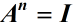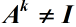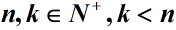# 特殊线性群SL(2,R)的有限Abelian子群Special Linear Group SL(2,R) of Finite Abelian Subgroup

DOI: 10.12677/AAM.2017.64073, PDF, , XML, 下载: 742  浏览: 2,387

Abstract: In this paper, we study the finite Abelian subgroup of special linear group SL(2,R)  by using the eigenvalue theory of λ-matrix and the solution method of constant coefficient linear homogeneous recursive relations. We get the structure of cyclic group for arbitrary order n of SL(2,R) , that is to say, all the solutions of the 2 order matrix equation:and() are given out. Furthermore, we hope to determine the structure of finite Abelian subgroup of special linear group SL(2,R) by discussing the commutativity between generators of arbitrary order cyclic group.

  唐高华. 近世代数[M]. 北京: 清华大学出版社, 2008.  姚慕生, 吴泉水. 高等代数学[M]. 第2版. 上海: 复旦大学出版社, 2008.  杨振声. 组合数学及其算法[M]. 第3版. 合肥: 中国科学技术大学出版社, 2006.  刘军成. GL(2, Z[i])的有限交换子群[J]. 湖北大学学报(自然科学版), 2010, 32(2): 233-240.